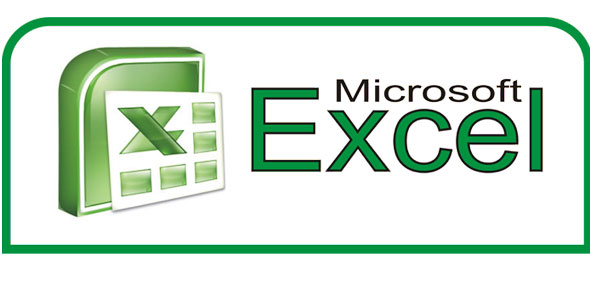# Microsoft Excel 2007 Basics 2

9 Questions | Total Attempts: 376SettingsCreate your own QuizThis quiz will measure your understanding of Excel 2007, part 2 of the basics

• 1.
What is a Chart?
• A.

Also known as a graph, it is a visual way to display and compare data

• B.

A way of arranging data in a particular order

• C.

A calculation that describes the relationship between cells

• 2.
A  worksheet is..
• A.

A symbol that represents a specific action

• B.

Refers to the change a formula makes when it is moved or copied to other cells with different data

• C.

A table of data that is organized into rows and columns

• 3.
What is a Formula?
• A.

A calculation that describes the relationship between cells

• B.

Excel automatically knows that you want to add the column of numbers above or add the row of numbers to the left

• C.

This is what rows are sorted by

• 4.
A Range in Microsoft Excel refers to...
• A.

A group of cells

• B.

A calculation that describes the relationship between cells

• C.

A way of arranging data in a particular order

• 5.
If you sort your information in an  Ascending sort you are?
• A.

A sort to rearrange data from highest to lowest, such as Z to A or largest number to smallest

• B.

A sort to rearrange data from lowest to highest, such as A to Z or smallest number to largest

• C.

Another way to reduce the amount of information displayed

• 6.
A Function is?
• A.

A symbol that represents a specific action

• B.

A way of arranging data in a particular order

• C.

A built-in formula in Excel

• 7.
What is a Workbook?
• A.

A table of data that is organized into rows and columns

• B.

The Excel file that holds your worksheets

• C.

A built-in formula in Excel

• 8.
What is the Criteria  function in excel?
• A.

A sort to rearrange data from lowest to highest, such as A to Z or smallest number to largest

• B.

This is what rows are sorted by

• C.

Another way to reduce the amount of information displayed

• 9.
What is the function of the AutoFit ?
• A.

A symbol that represents a specific action

• B.

A way of arranging data in a particular order

• C.

Automatically change the width of a column to fit the longest entry or change the height of a row to fit the font

Related TopicsBack to top
×

Wait!
Here's an interesting quiz for you.